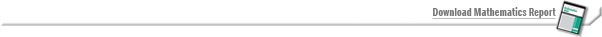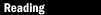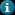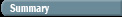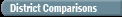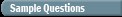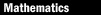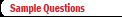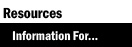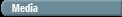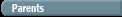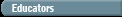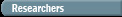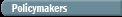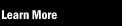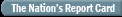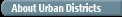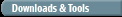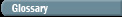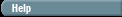Sample Questions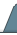Reading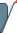Mathematics2005 NAEP Mathematics Sample QuestionsThe sample questions below give a brief introduction to the materials that assess the mathematical ability of students in grades 4 and 8. All questions are based on the NAEP mathematics framework, which defines the test design and content. The framework was developed through an extensive national consensus process and adopted by the National Assessment Governing Board (NAGB). Visit the NAGB website to download the full text of the mathematics framework.

Several other tools are available to help students, parents, and teachers look more closely at NAEP questions. View the item maps that provide concrete examples of what students at various achievement levels likely know and can do. Visit the NAEP Questions Tool to see over 1,600 items in all NAEP subject areas.

Sample Grade 4 Short Constructed-Response Question

Sample Question 1 is a short constructed-response question in the number properties and operations content area. This question asked students to identify the point indicated on a number line.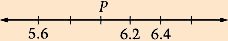1. On the number line above, what number would be located at
point P?

56 percent of fourth-graders wrote responses rated as "Correct."

Sample Question 2 is a multiple-choice question in the algebra content area. This question asked students to represent a given situation with an algebraic expression.

2. N stands for the number of hours of sleep Ken gets each night.
Which of the following represents the number of hours of sleep
Ken gets in 1 week?

 A) N + 7 B) N - 7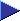C) N x 7 D) N ÷ 7

Sample Grade 8 Short Constructed-Response Question

Sample Question 3 is a short constructed-response question in the geometry content area. This question asked students to shade 5 additional squares in a grid that has 3 shaded squares to create a symmetric pattern. Students were given paper squares for this question.

3. Shade five more squares on the grid below so that if your
completed figure were folded along the fold line both sides
would match.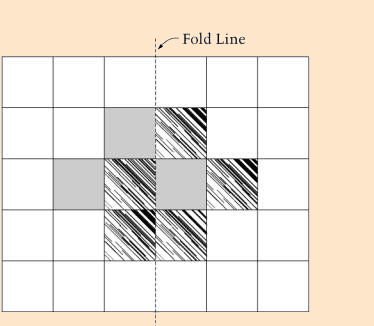58 percent of eighth-graders wrote responses rated as "Correct."

 A) 40 B) 100C) 121 D) 144 E) 169